Journal of Vibroengineering

Published: 07 July 2022

# Vibration characteristics of mistuned multistage bladed disks of the aero-engine compressor

Hongyuan Zhang1
Haiyu Feng2
Xiaomei You3
Tianyu Zhao4
1School of Automotive and Transportation, Shenyang Ligong University, Shenyang, China
2, 3School of Mechanical Engineering, Shenyang Ligong University, Shenyang, China
4College of Sciences, Northeastern University, Shenyang, China
Corresponding Author:
Hongyuan Zhang
Views 94

#### Abstract

In order to analyze the vibration characteristics of mistuned multistage bladed disks of an aero-engine compressor, a finite element reduction model of mistuned multistage bladed disks is established based on substructure modal synthesis method. The accuracy of the substructure model was verified by comparing calculation accuracy of the substructure model and the integral model. The influence of different modal truncation numbers on the calculation results are discussed. The vibration modes of each stage of the bladed disks are obtained, the forced response is analyzed from the perspective of strain energy. The result shows that modal truncation number, rotation softening effect, and speed have significant effects on the dynamic frequency calculation results of the multistage bladed disks. The typical mode shapes of the first 200 orders of multistage bladed disks are obtained. With the increase of mistuning standard deviation, the strain energy of multistage bladed disk system decreases gradually.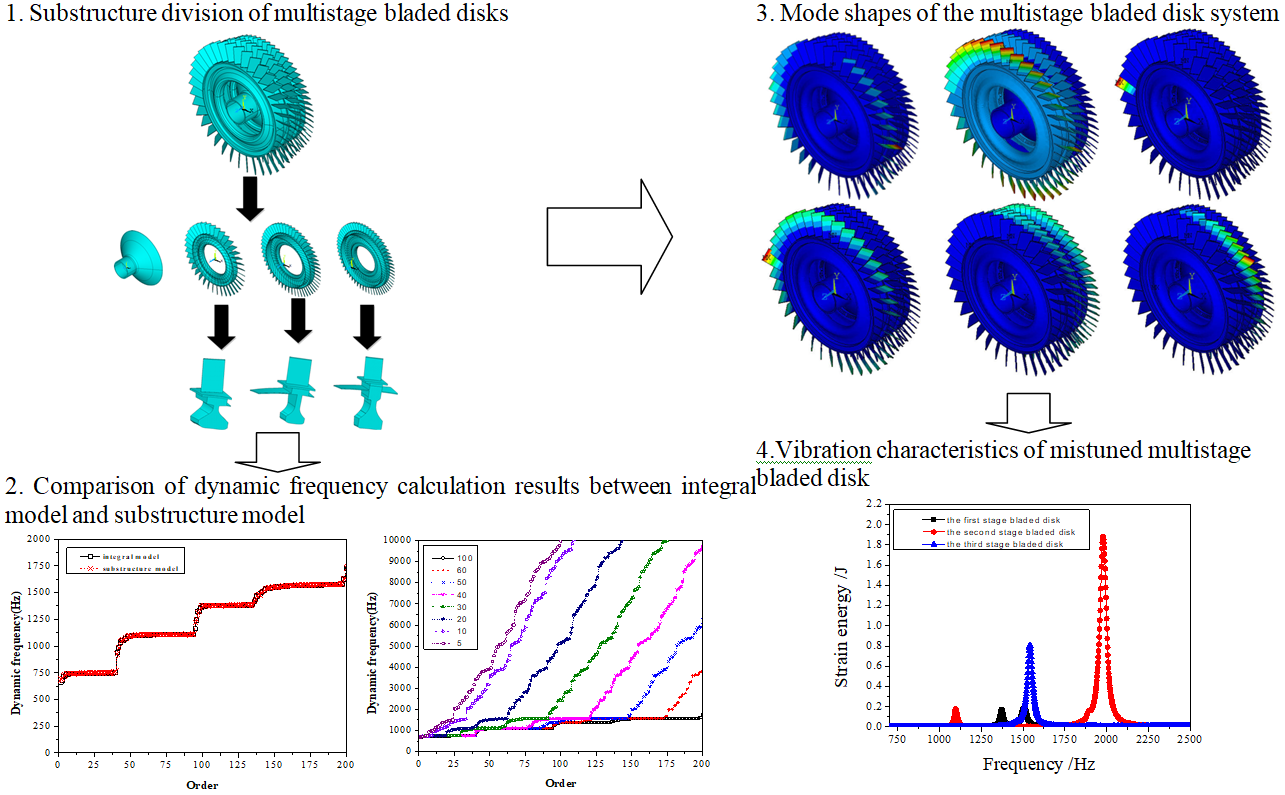#### Highlights

• The finite element reduction model of multistage bladed disk was established
• The modal shapes of typical orders of multistage bladed disks are obtained.
• The vibration of the mistuned bladed disk is analyzed from the perspective of strain energy.

## 1. Introduction

The aero-engine compressor is composed of multistage disks. Interstage coupling controls the energy propagation between the disks and is regarded as the main factor leading to the difference dynamic performance between single-stage and multistage disks. However, the influence of interstage coupling is usually ignored in mistuned vibration analysis of single-stage disks [1-3].

Most of the above scholars' research on the multistage bladed disk focuses on the modal vibration characteristics of the secondary disk. The models used are Lumped parameter model or cantilever beam model, and the finite element model of the multistage bladed disk system is not used. This paper based on the substructure modal synthesis method, a finite element reduction model of mistuned multistage bladed disk is established, and the accuracy of the model is verified. The vibration characteristics of mistuned multistage bladed disk are obtained through modal analysis and forced response analysis.

## 2. Substructure modal synthesis method

In order to reduce the calculation scale of the bladed disk system, improve the calculation and analysis efficiency, it is necessary to reduce the degrees of freedom of the mistuned multistage bladed disks. The structural dynamic equation can be expressed as:

1
$\begin{array}{ll}{\left[M\right]}_{i}{\left\{\stackrel{¨}{X}\right\}}_{i}+{\left[C+G\right]}_{i}{\left\{\stackrel{˙}{X}\right\}}_{i}+{\left[K+{K}_{\mathrm{c}}+{K}_{S}\right]}_{i}{\left\{X\right\}}_{i}={\left\{F\right\}}_{i},& \left(i=1,2,\cdots ,n\right)\end{array},$

where $M$, $C$, $G$, $K$, ${K}_{\mathrm{c}}$, ${K}_{S}$, $F$, $X$ and $n$ are mass matrix, damping matrix, Coriolis force matrix, the stiffness matrix of the structure, centrifugal stiffening effect matrix of rotating blades, rotation softening matrix, force acting on the substructure model, displacement vector, and number of blades respectively.

The general forced vibration equation of the $i$-th substructure finite element reduced order models can be expressed as:

2
$\begin{array}{ll}{\left[M\right]}_{i}{\left\{\stackrel{¨}{X}\right\}}_{i}+{\left[C\text{'}\right]}_{i}{\left\{\stackrel{˙}{X}\right\}}_{i}+{\left[K\text{'}\right]}_{i}{\left\{X\right\}}_{i}={\left\{F\right\}}_{i},& \left(i=1,2,\cdots ,n\right)\end{array},$

where $C\text{'}=C+G$, $C$ is damping matrix, and $G$ is Coriolis force matrix, where $C$, $\alpha$ and $\beta$ are given by:

3
$C=\alpha M+\beta K,$
4
$\left\{\begin{array}{l}\alpha =\frac{4\pi {f}_{n1}{f}_{n2}\left({f}_{n1}{\xi }_{2}-{f}_{n2}{\xi }_{1}\right)}{{f}_{n1}^{2}-{f}_{n2}^{2}},\\ \beta =\frac{{f}_{n2}{\xi }_{2}-{f}_{n1}{\xi }_{1}}{\pi \left({f}_{n2}^{2}-{f}_{n1}^{2}\right)},\end{array}\right\$

where ${f}_{n1}$and ${f}_{n2}$ are the first-order and second-order natural frequencies, respectively, ${\xi }_{1}$ and ${\xi }_{2}$ are the corresponding modal damping ratios.

The Coriolis force matrix obtained as:

5
$G=2\sum _{1}^{n}{\int }_{V}{N}^{T}\mathrm{\Omega }NdV,$

where $N$ is the shape function matrix; n is the total number of elements; $\mathrm{\Omega }$ is the rotational matrix. The rotational matrix $\mathrm{\Omega }$ obtained as:

6
$\mathrm{\Omega }=\left[\begin{array}{ccc}0& -{\mathrm{\Omega }}_{z}& {\mathrm{\Omega }}_{y}\\ {\mathrm{\Omega }}_{z}& 0& -{\mathrm{\Omega }}_{x}\\ -{\mathrm{\Omega }}_{y}& {\mathrm{\Omega }}_{x}& 0\end{array}\right],$
7
${K}^{\mathrm{\text{'}}}=K+{K}_{\mathrm{c}}+{K}_{S},$

where $K$ is the stiffness matrix of the structure, ${K}_{c}$ is the centrifugal stiffening effect matrix of the rotating blades, and ${K}_{S}$ is the rotation-softening matrix.

The displacement vector $X$ is composed of the degree of freedom ${X}_{m}$ at the boundary and the internal freedom ${X}_{s}$ of the non-interface, that is:

The Eq. (2) can be presented as below:

8
${\left[\begin{array}{ll}{M}_{mm}& {M}_{ms}\\ {M}_{sm}& {M}_{ss}\end{array}\right]}_{i}{\left\{\begin{array}{l}{\stackrel{¨}{X}}_{m}\\ {\stackrel{¨}{X}}_{s}\end{array}\right\}}_{i}+{\left[\begin{array}{ll}{C\text{'}}_{mm}& {C\text{'}}_{ms}\\ {C\text{'}}_{sm}& {C\text{'}}_{ss}\end{array}\right]}_{i}\left\{\begin{array}{c}{\stackrel{˙}{X}}_{m}\\ {\stackrel{˙}{X}}_{s}\end{array}\right\}+{\left[\begin{array}{ll}{K\text{'}}_{mm}& {K\text{'}}_{ms}\\ {K\text{'}}_{sm}& {K\text{'}}_{ss}\end{array}\right]}_{i}{\left\{\begin{array}{c}{X}_{m}\\ {X}_{s}\end{array}\right\}}_{i}={\left\{\begin{array}{c}{f}_{m}\\ 0\end{array}\right\}}_{i},$

where, ${f}_{m}$ is the interface force. Let the substructure of the interface to be fixed, even if ${X}_{m}=0$, it is possible to obtain as follows:

9
${\left[{M}_{ss}\right]}_{i}{\left\{{\stackrel{¨}{X}}_{s}\right\}}_{i}+{\left[{C\text{'}}_{ss}\right]}_{i}{\left\{{\stackrel{˙}{X}}_{s}\right\}}_{i}+{\left[{K\text{'}}_{ss}\right]}_{i}{\left\{{X}_{s}\right\}}_{i}=0.$

From this, the normalized mode $\left[{\varphi }_{N}\right]$ is solved, that is $\left[{\varphi }_{N}\right]=\left[{\varphi }_{l},{\varphi }_{h}\right]$, where, $\left[{\varphi }_{l}\right]$ – low order modal, $\left[{\varphi }_{h}\right]$ – high order modal.

This modal set satisfies the following conditions:

10
${\left[{\varphi }_{N}\right]}^{T}\left[{M}_{ss}\right]\left[{\varphi }_{N}\right]=E,$
11
${\left[{\varphi }_{N}\right]}^{T}\left[{K\text{'}}_{ss}\right]\left[{\varphi }_{N}\right]=\left[\mathrm{\Lambda }\right]=\left[\begin{array}{ll}{\mathrm{\Lambda }}_{l}& \\ & {\mathrm{\Lambda }}_{h}\end{array}\right],$

where, $\left[{\mathrm{\Lambda }}_{l}\right]=\mathrm{d}\mathrm{i}\mathrm{a}\mathrm{g}\left({\lambda }_{1}^{2},{\lambda }_{2}^{2},\cdots ,{\lambda }_{l}^{2}\right)$, $\left[{\mathrm{\Lambda }}_{h}\right]=\mathrm{d}\mathrm{i}\mathrm{a}\mathrm{g}\left({\lambda }_{l+1}^{2},{\lambda }_{l+2}^{2},\cdots ,{\lambda }_{h}^{2}\right)$, $E$ is a identity matrix.

When the higher order mode sets are omitted, and the lower order mode set ${\varphi }_{l}$ is selected to form the master modal set ${\varphi }_{m}$ of the substructures, that is:

12
$\left[{\varphi }_{m}\right]=\left[{\varphi }_{l}\right].$

If the fixed interface method is used, ${\varphi }_{J}$ can be expressed as:

$\left[{\varphi }_{J}\right]=-{{\left[{{K}^{\text{'}}}_{ss}\right]}^{-1}}_{i}{\left[{{K}^{\text{'}}}_{sm}\right]}_{i}\left[E\right]=-{{\left[{{K}^{\text{'}}}_{ss}\right]}^{-1}}_{i}{\left[{{K}^{\text{'}}}_{sm}\right]}_{i}.$

Definition $\left[{\varphi }_{c}\right]$ is the constrained modal set:

13
$\left[{\varphi }_{c}\right]=\left[\begin{array}{l}E\\ {\varphi }_{J}\end{array}\right]=\left[\begin{array}{c}E\\ -{{K\text{'}}_{ss}}^{-1}{K\text{'}}_{sm}\end{array}\right].$

The Ritz base vector of the $i$ substructure:

14
$\left[\varphi \right]=\left[{\varphi }_{m},{\varphi }_{c}\right]=\left[\begin{array}{cc}0& E\\ {\varphi }_{l}& {\varphi }_{J}\end{array}\right].$

Coordinate transformation is:

15
$\left[X\right]=\left[\varphi \right]\left[p\right]=\left[\begin{array}{cc}0& E\\ {\varphi }_{l}& {\varphi }_{J}\end{array}\right]\left[\begin{array}{c}{p}_{m}\\ {p}_{s}\end{array}\right].$

Through the Eq. (15), it can achieve the transformation of the equation of motion from physical coordinates to modal coordinates:

16
${\left[\stackrel{-}{M}\right]}_{i}{\left\{\stackrel{¨}{X}\right\}}_{i}+{\left[\stackrel{-}{C}\right]}_{i}{\left\{\stackrel{˙}{X}\right\}}_{i}+{\left[\stackrel{-}{K}\right]}_{i}{\left\{X\right\}}_{i}={\left\{\stackrel{-}{F}\right\}}_{i},$

where:

The coordinate transformation of Eq. (15), which uses only low-order mode and ignores the high-order mode, that is used to adapt the mode truncation, so the node degree of freedom is greatly reduced, will be made for obtaining the $i$ substructure formula deduced from the Eq. (15):

17
$\begin{array}{l}\left[\begin{array}{llll}{\stackrel{-}{M}}_{1}& & & 0\\ & {\stackrel{-}{M}}_{2}& & \\ & & \ddots & \\ 0& & & {\stackrel{-}{M}}_{i}\end{array}\right]\left\{\begin{array}{l}{\stackrel{¨}{p}}_{1}\\ {\stackrel{¨}{p}}_{2}\\ ⋮\\ {\stackrel{¨}{p}}_{i}\end{array}\right\}+\left[\begin{array}{llll}{\stackrel{-}{C}}_{1}& & & 0\\ & {\stackrel{-}{C}}_{2}& & \\ & & \ddots & \\ 0& & & {\stackrel{-}{C}}_{i}\end{array}\right]\left\{\begin{array}{l}{\stackrel{˙}{p}}_{1}\\ {\stackrel{˙}{p}}_{2}\\ ⋮\\ {\stackrel{˙}{p}}_{i}\end{array}\right\}+\left[\begin{array}{llll}{\stackrel{-}{K}}_{1}& & & 0\\ & {\stackrel{-}{K}}_{2}& & \\ & & \ddots & \\ 0& & & {\stackrel{-}{K}}_{i}\end{array}\right]\left\{\begin{array}{l}{p}_{1}\\ {p}_{2}\\ ⋮\\ {p}_{i}\end{array}\right\}=\left\{\begin{array}{l}{\stackrel{-}{F}}_{1}\\ {\stackrel{-}{F}}_{2}\\ ⋮\\ {\stackrel{-}{F}}_{i}\end{array}\right\}\\ \left(i=1,2,\cdots ,n\right).\end{array},$

If the rigid connection interface is considered, through the force balance and displacement coordination condition, it is possible to obtain as follows:

18
$\begin{array}{ll}\left\{{X}_{m1}\right\}=\left\{{X}_{m2}\right\}=\cdots =\left\{{X}_{mi}\right\},& \left(i=1,2,\cdots ,n\right)\end{array},$
19
$\begin{array}{ll}\left\{{f}_{m1}\right\}+\left\{{f}_{m2}\right\}+\cdots +\left\{{f}_{mi}\right\}=0,& \left(i=1,2,\cdots ,n\right)\end{array}.$

The non-independent coordinate can be transformed into the equation of motion of the generalized coordinate $\left\{q\right\}$ in the following form:

20
$\left\{p\right\}=\left\{\begin{array}{c}\begin{array}{l}{p}_{m1}\\ {p}_{s1}\end{array}\\ \begin{array}{l}{p}_{m2}\\ {p}_{s2}\end{array}\\ \begin{array}{l}⋮\\ ⋮\\ {p}_{mi}\end{array}\\ {p}_{si}\end{array}\right\}=\left[\begin{array}{llllll}E& 0& 0& \cdots & 0& 0\\ 0& E& 0& \cdots & 0& 0\\ E& 0& 0& \cdots & 0& 0\\ 0& 0& E& \cdots & 0& 0\\ E& 0& 0& \cdots & 0& 0\\ ⋮& ⋮& ⋮& \ddots & 0& 0\\ E& 0& 0& 0& 0& 0\\ 0& 0& 0& 0& 0& E\end{array}\right]\left\{\begin{array}{c}{q}_{m}\\ {q}_{s1}\\ {q}_{s2}\\ ⋮\\ {q}_{si}\end{array}\right\}.$

That is:

21
$\left\{p\right\}=\left[\beta \right]\left\{q\right\}.$

The Eq. (21) is transformed into the equation of motion of the generalized coordinate by using this form $\left\{q\right\}$:

22
$\begin{array}{ll}{\left[\stackrel{~}{M}\right]}_{i}{\left\{\stackrel{¨}{q}\right\}}_{i}+{\left[\stackrel{~}{C}\right]}_{i}{\left\{\stackrel{˙}{q}\right\}}_{i}+{\left[\stackrel{~}{K}\right]}_{i}{\left\{q\right\}}_{i}={\left\{\stackrel{~}{F}\right\}}_{i},& \left(i=1,2,\cdots ,38\right)\end{array},$

where, ${\left[\stackrel{~}{M}\right]}_{i}={\left[\beta \right]}^{T}{\left[\stackrel{-}{M}\right]}_{i}\left[\beta \right]$, ${\left[\stackrel{~}{K}\right]}_{i}={\left[\beta \right]}^{T}{\left[\stackrel{-}{K}\right]}_{i}\left[\beta \right]$, ${\left[\stackrel{~}{C}\right]}_{i}={\left[\beta \right]}^{T}{\left[\stackrel{-}{C}\right]}_{i}\left[\beta \right]$, ${\left[\stackrel{~}{F}\right]}_{i}={\left[\beta \right]}^{T}{\left[\stackrel{-}{F}\right]}_{i}$.

The Eq. (22) can be adopted to solve the natural frequency of the system and the vibration mode under the generalized coordinates $\left\{q\right\}$. The vibration mode under the generalized coordinates can be returned to the physical coordinates by the coordinate transformation from Eq. (21) and form transformation from Eq. (15), thus the vibration mode of the physical system coordinates can be obtained.

Fig. 1Substructure division process of multistage bladed disks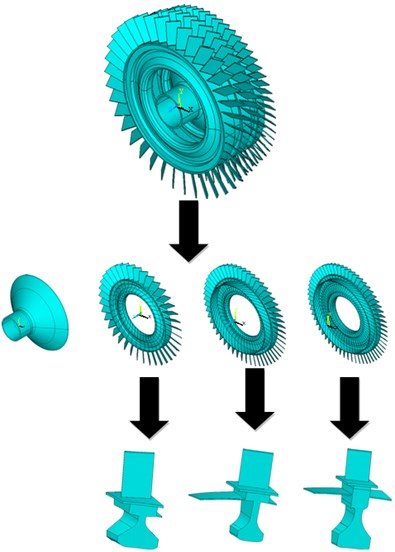The finite element model of three-stage bladed disk is based on ANSYS 15.0. A three-stage bladed disks are divided into substructures is shown in Fig. 1. So as to model of three-stage bladed disk in ANSYS, solid185 elements are used. The mechanical properties of the three-stage bladed disk is shown in Table 1.

Table 1The mechanical properties of the three-stage bladed disk

 Blade material property Property value Disk material property Property value Mass density 4400kg⋅m−3 Mass density 8200kg⋅m−3 Modulus of elasticity 114 GPa Modulus of Elasticity 166 GPa Poisson’s ratio 0.3 Poisson’s ratio 0.3

The blade of each stage bladed disk and disk connected with it are divided into one substructure. The first stage bladed disk is divided into 38 substructures, the second stage bladed disk is divided into 52 substructures, and the third stage bladed disk is divided into 60 substructures. The finite element model of the three-stage bladed disk system after the substructure integrated condensed super element is shown in Fig. 2.

Fig. 2Finite element model of multistage bladed disks after condensing super element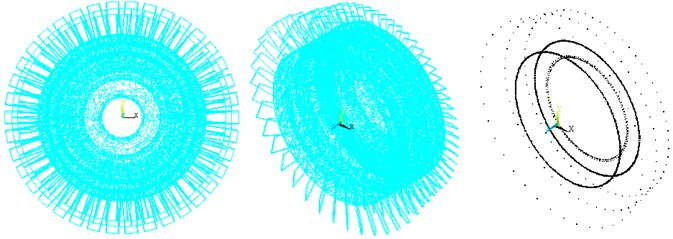## 3. Modal analysis

In this section, finite element analysis is used o obtain the natural frequency and mode shape, ANSYS package (Released version 15.0) is used. Through the modal analysis between the integral model and the substructure model, the first 500 natural frequencies and vibration modes are obtained respectively, and compared to verify the accuracy of the substructure model and determine the appropriate modal truncation number. The block diagram of modal analysis is shown in Fig. 3.

The aero-engine compressor bladed disks system is a high-speed rotating power system. Whether the effect of rotating speed is considered will affect the accuracy of the dynamic analysis. As shown in Fig. 4 and Table 2, the natural frequency calculation comparison between the integral bladed disks model and the substructure model is shown. The effect caused by the rotation of the bladed disk is considered in the calculation, and the dynamic frequency of the bladed disks system is obtained. It can be seen from the figure that the substructure model is in good agreement with the calculation results of the integral blade disk model within 225 order, which shows that the substructure model still has high calculation accuracy and can meet the calculation requirements on the premise of ignoring the high-order modes, reducing the number of degrees of freedom of the model and improving the calculation speed.

In order to verify the accuracy of the calculation results of the substructure model, the mode shapes of the integral bladed disks model and the substructure model are compared, and the comparison results are shown in Fig. 5. It can be seen from the figure that the vibration modes of the substructure under each mode are consistent with the calculation results of the integral bladed disk model, and the calculation accuracy of the substructure model is high, which can meet the requirements of the next dynamic calculation.

Fig. 3The block diagram of modal analysis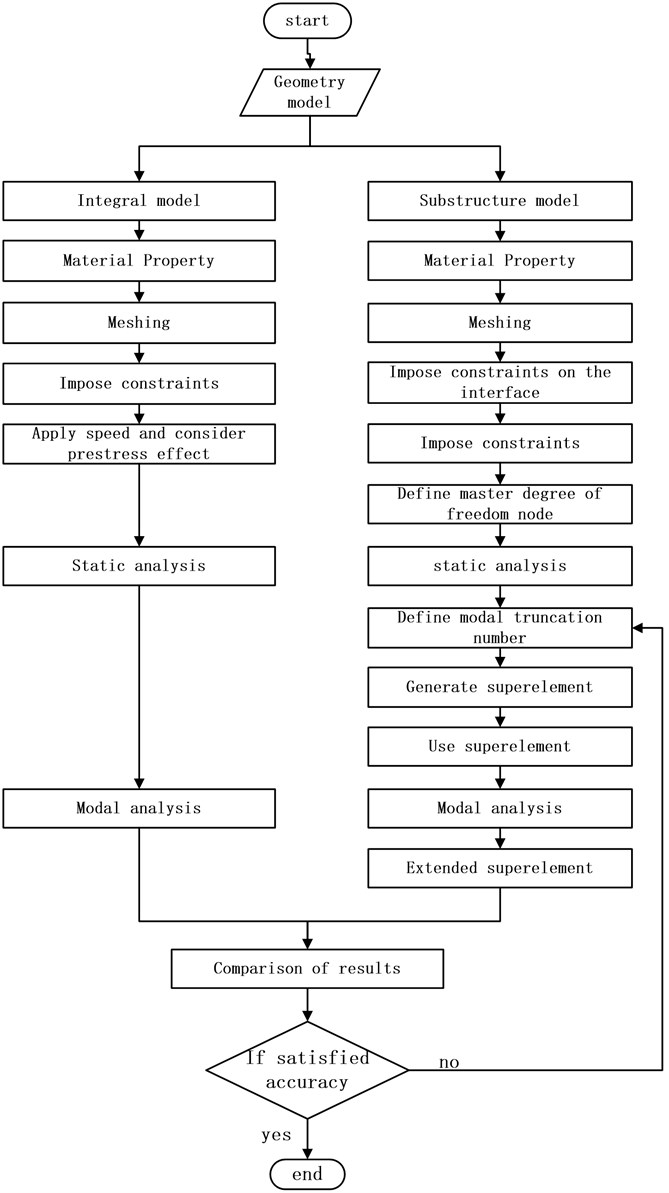Fig. 4Comparison of dynamic frequency calculation results between integral model and substructure model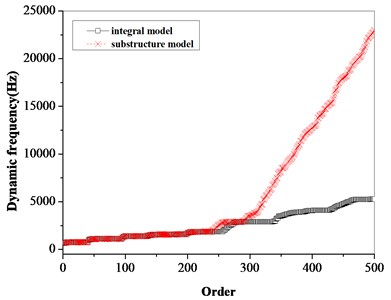a) 500 order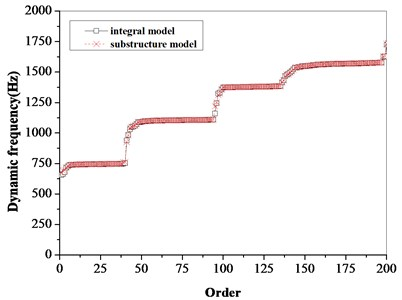b) 200 order

Table 2Comparison of dynamic frequency calculation results between integral model and substructure model

 Order Natural frequency (Hz) Absolute error (%) Integral model Substructure model 1 655.07 673.62 –0.02832 2 662.14 691.52 –0.04437 3 679.52 695.56 –0.0236 4 715.88 717.13 –0.00175 5 724.64 726.69 –0.00283 6 737.13 737.05 0.000109 7 738.29 738.07 0.000298 8 739.4 739.49 –0.00012 9 740.03 739.82 0.000284 10 741.11 740.84 0.000364 11 741.57 741.29 0.000378 12 742.01 742.03 –2.7E-05 13 742.55 742.42 0.000175 14 742.69 742.6 0.000121 15 742.92 742.8 0.000162 16 743.07 742.98 0.000121 17 743.35 743.19 0.000215 18 743.43 743.48 –6.7E-05 19 743.55 743.95 –0.00054 20 743.83 744.03 –0.00027 21 744.32 744.14 0.000242 22 744.63 744.29 0.000457 23 744.71 744.58 0.000175 24 744.92 744.62 0.000403 25 745 744.64 0.000483 26 745.07 744.88 0.000255 27 745.11 745.11 0 28 745.56 745.26 0.000402 29 745.62 745.31 0.000416 30 745.69 745.35 0.000456 31 745.75 745.55 0.000268 32 745.77 745.57 0.000268 33 746.12 745.75 0.000496 34 746.29 745.83 0.000616 35 746.44 745.88 0.00075

Fig. 5Comparison of modal shapes between integral model and substructure model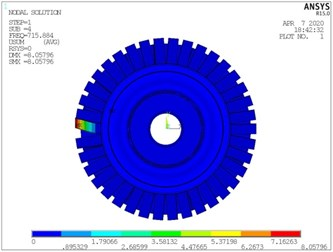a) The fourth order of integral model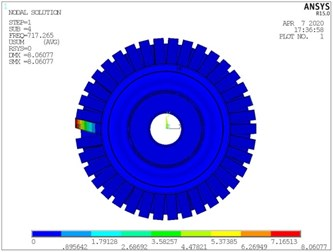b) The fourth order of substructure model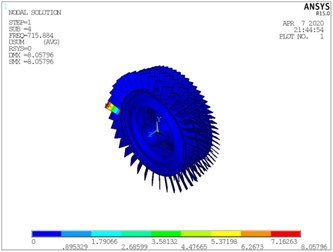c) The fourth order of integral model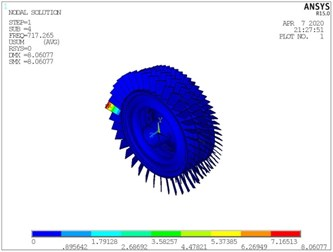d) The fourth order of substructure model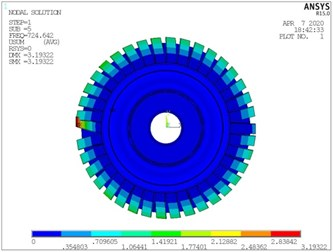e) The fifth order of integral model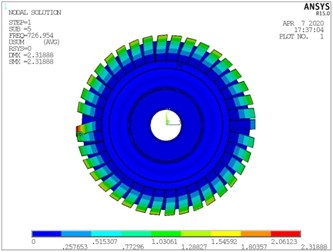f) The fifth order of substructure model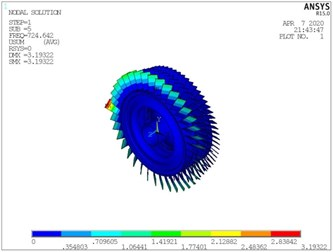g) The fifth order of integral model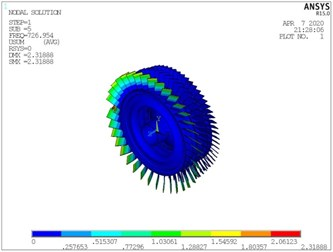h) The fifth order of substructure model

The basic idea of degree of freedom reduction by substructure modal synthesis method is to ignore the high-order modes and intercept the low-order modes. On the premise of meeting the calculation accuracy of the low-order model, the number of degrees of freedom of the dynamic model is reduced by selecting the appropriate mode truncation number, so as to improve the calculation speed. Fig. 6 shows the dynamic frequency comparison of the three-stage bladed disk system when different modal truncation numbers are selected. It can be seen from the figure that the accuracy of low-order modal calculation increases with the increase of modal truncation number. From Fig. 6(b), when the modal truncation number is greater than 30, the first 25 modal calculation results can meet the requirements. When the modal truncation number is greater than 60, the accuracy of the first 175 modal results is higher.

Fig. 6Effect of different modal truncation numbers on dynamic frequency of multistage bladed disk system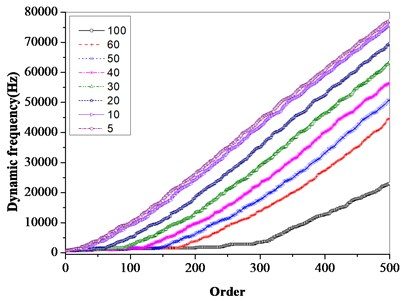a) 500 order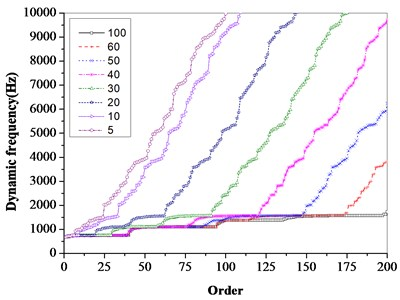b) 200 order

For rotating machinery, the rotary softening effect has a certain impact on the dynamics of the bladed disk system. The rotary softening effect is considered in the calculation of multistage bladed disks, and the calculation results shown in Fig. 7 are obtained. It can be seen from the figure that the rotating software effect has a certain impact on the dynamic characteristics of the bladed disk system.

Fig. 7Effect of rotary softening on dynamic frequency of bladed disks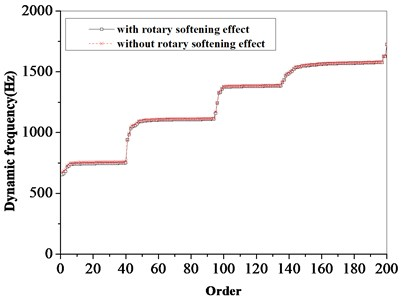a) Integral model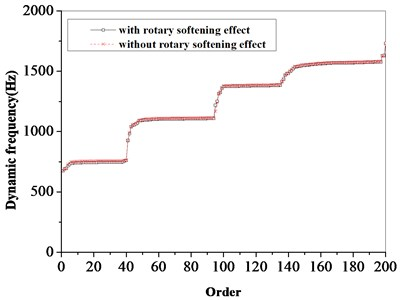b) Substructure model

The rotating speed of the bladed disk system has a certain impact on the dynamic frequency of the bladed disk system. It can be seen from Table 3 and Fig. 8 that the dynamic frequency gradually increases with the increase of rotating speed.

The first 200 order dynamic frequencies and mode shapes of multistage bladed disk system are shown in Table 4.

Table 3Effect of rotating speed on dynamic frequency of bladed disks

 Order Speed rad/s 795.8 954.9 1114.1 1188.3 1273.2 1432.4 1 607.49 632.59 660.58 673.86 685.53 687.02 2 650.31 671.85 688.52 692.8 697.57 696.34 3 662.54 679.43 693.04 696.82 700.07 729.1 4 664.15 682.56 704.67 717.27 732.62 764.37 5 666.3 688.64 714.12 726.95 742.09 772.93 6 674.65 697.64 723.85 737.06 752.67 784.49 7 675.57 698.59 724.84 738.07 753.71 785.59 8 676.92 699.97 726.27 739.53 755.2 787.17 9 677.14 700.23 726.57 739.85 755.55 787.56 10 678.03 701.15 727.53 740.84 756.56 788.63 11 678.47 701.6 727.98 741.3 757.02 789.11 12 679.16 702.3 728.71 742.03 757.77 789.89 13 679.5 702.66 729.09 742.42 758.17 790.31 14 679.65 702.82 729.26 742.6 758.36 790.52 15 679.83 703.01 729.46 742.8 758.56 790.73 16 680.07 703.22 729.65 742.98 758.73 790.88 17 680.22 703.39 729.84 743.19 758.96 791.13 18 680.5 703.68 730.13 743.48 759.25 791.43 19 681.01 704.17 730.61 743.95 759.72 791.89 20 681.05 704.23 730.68 744.03 759.8 791.99 21 681.14 704.33 730.79 744.14 759.91 792.11 22 681.37 704.53 730.96 744.29 760.05 792.2 23 681.66 704.84 731.26 744.58 760.31 792.37 24 681.69 704.85 731.28 744.62 760.39 792.55 25 681.73 704.87 731.3 744.64 760.41 792.59 26 681.92 705.09 731.54 744.88 760.65 792.82 27 682.19 705.35 731.78 745.12 760.87 793.02 28 682.32 705.48 731.92 745.26 761.02 793.19 29 682.38 705.54 731.97 745.31 761.07 793.24 30 682.42 705.58 732.01 745.35 761.11 793.27 31 682.66 705.81 732.22 745.55 761.3 793.42 32 682.67 705.82 732.24 745.57 761.32 793.48 33 682.85 706 732.42 745.75 761.51 793.65 34 682.92 706.07 732.5 745.83 761.59 793.75 35 682.96 706.11 732.54 745.88 761.64 793.8

Fig. 8Effect of rotating speed on dynamic frequency of bladed disks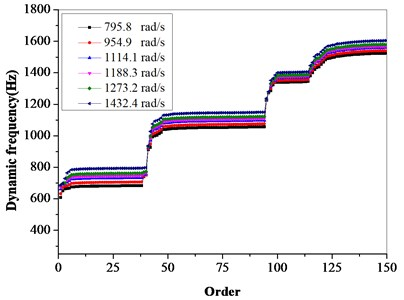It can be seen from Table 4 that the vibration of the first 40 stage bladed disks system is mainly concentrated in the first stage bladed disk, the vibration mode is mainly the first-order bending vibration of the blades. The 41st and 42nd modes are mainly the coupled vibration of the second-stage and third-stage blades. The first-order bending vibration of the second stage blades is the main mode of 43th-94th. the 99th-135th vibration modes are mainly the first-order torsion vibration of the first stage blades. the 136th-197thare the first-order bending vibration of the third stage blades. the 198th-200th are the coupled vibration of the three-stage blades and disks.

Table 4First 200th order dynamic frequencies and mode shapes of the multistage bladed disk system

 Order number Dynamic frequency/Hz Vibration mode Mode pattern of a typical order 1 655.073 First-order bending vibration of the first-stage blades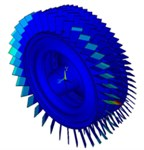2 662.144 Coupled vibration of the first-stage and second-stage blades and disks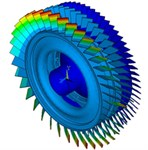3-4 679.515-715.884 First-order bending vibration of the first-stage blades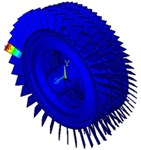5-40 724.642-751.635 First-order bending vibration of the first-stage blades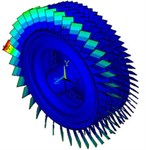41-42 938.904-982.939 Coupled vibration of the second-stage and third-stage blades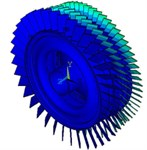43-94 1031.86-1109.85 First-order bending vibration of the second-stage blades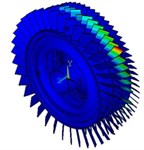95 1159.27 Coupled vibration of the first-stage and second-stage blades and disks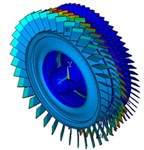96 1242.65 Coupled vibration of the three-stage blades and disks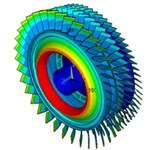97 1323.47 First-order blades torsion vibration of the first-stage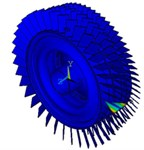98 1331.06 Coupled vibration of the three-stage blades and disks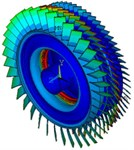99-135 1357.57-1385.17 First-order blade torsion vibration of the first-stage blades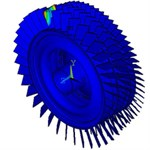136-197 1413.1-1577.73 First-order bending vibration of the third-stage blades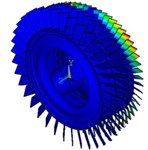198-200 1625.72-1724.05 Coupled vibration of the three-stage blades and disks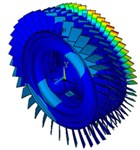## 4. Vibration characteristics of mistuned multistage bladed disks

The flow excitation acting on the compressor blades is often simply to a single point sinusoidal excitation acting on the blade tip. According to the modal vibration analysis results, the excitation frequency range is 650 Hz-2500 Hz. the mistuning value is normally distributed, and the vibration characteristics of multistage bladed disks with mistuning standard deviation of 1 % - 5 % are calculated.

Fig. 9 shows the amplitude frequency characteristics of the multistage bladed disks system. as can be seen from Fig. 9, the mistuning causes the amplitude peak resonance band of the second stage blades disk to widen. the amplitude peak of the second stage bladed disk increases gradually with the increase of mistuning standard deviation near 1980 Hz. Fig. 10 shows the maximum amplitude of each blade of multistage bladed disks system when the excitation frequency is 1980 Hz. the blades with large amplitude are concentrated in the second stage bladed disk. It can be seen from Table 5 that the mistuning increases the maximum vibration amplitude of the bladed disk system, the maximum vibration amplitude of bladed disk system occurs when the standard deviation of mistuning is 2 %.

Fig. 9Amplitude frequency characteristics of bladed disk system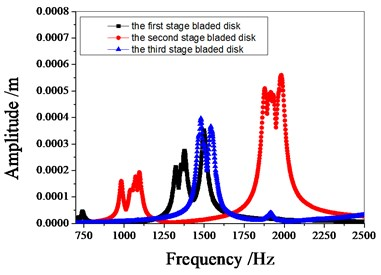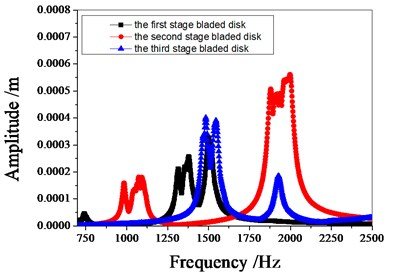b) 1 % mistuned bladed disk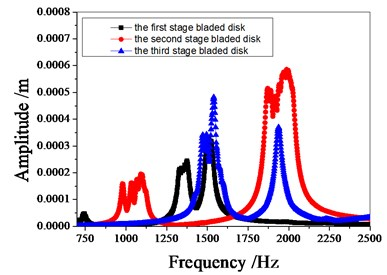c) 2 % mistuned bladed disk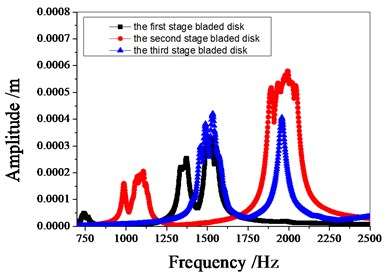d) 3 % mistuned bladed disk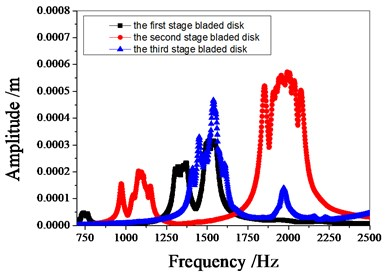e) 4 % mistuned bladed disk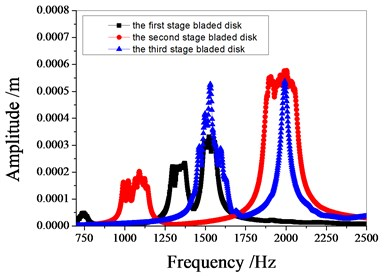f) 5 % mistuned bladed disk.

Fig. 10The maximum amplitude of each blades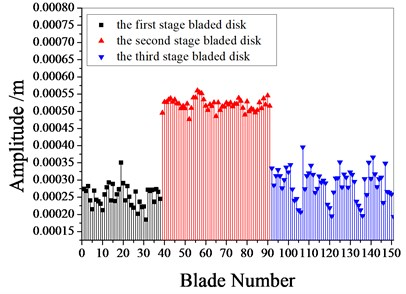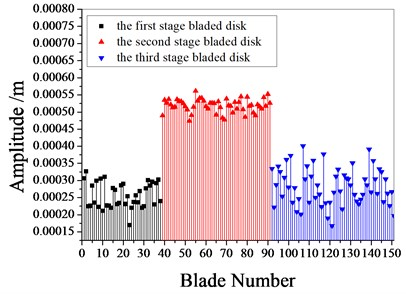b) 1 % mistuned bladed disk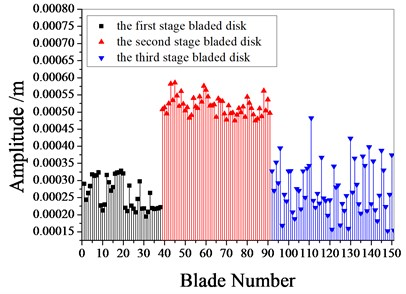c) 2 % mistuned bladed disk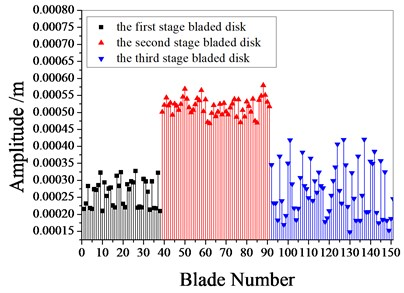d) 3 % mistuned bladed disk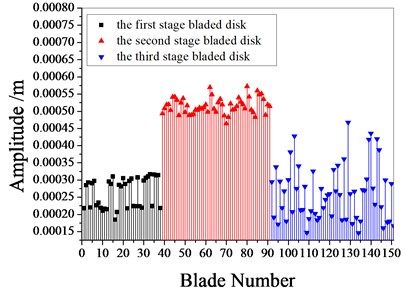e) 4 % mistuned bladed disk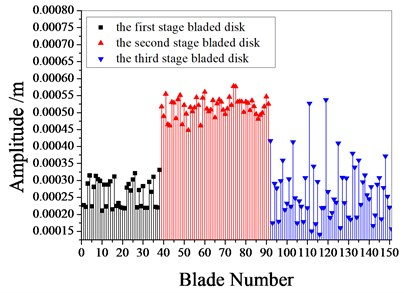f) 5 % mistuned bladed disk

Table 5The maximum amplitude of the multistage bladed disk system.

 Mistuning The maximum amplitude (×10-4 m) The first stage The second stage The third stage The maximum amplitude of bladed disk 0 % (tuned) 3.505 5.545 3.509 5.545 1% 3.269 5.61 3.772 5.61 2% 3.28 5.856 4.824 5.856 3% 3.238 5.688 4.207 5.688 4% 3.159 5.69 4.676 5.69 5% 3.312 5.777 4.173 5.777

Fig. 11Strain energy of bladed disk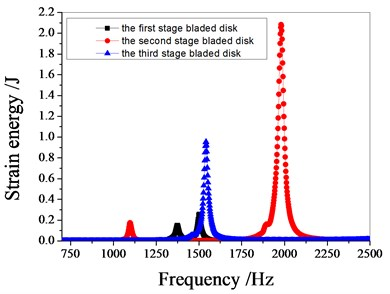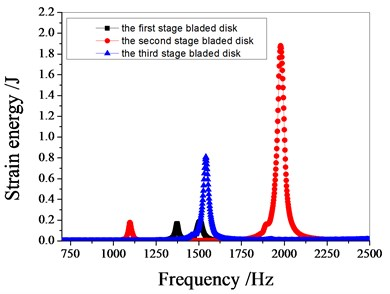b) 1 % mistuned bladed disk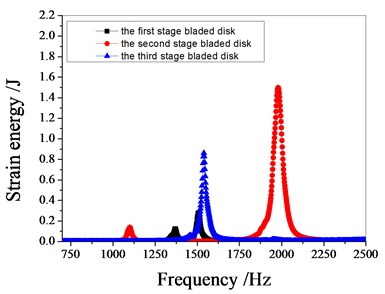c) 2 % mistuned bladed disk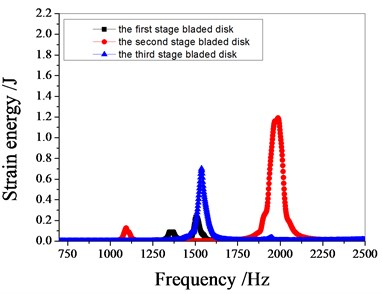d) 3 % mistuned bladed disk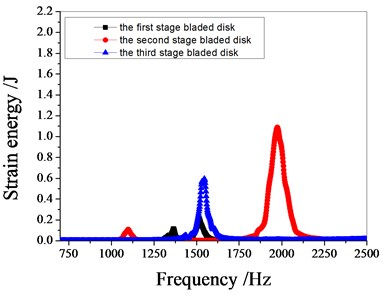e) 4 % mistuned bladed disk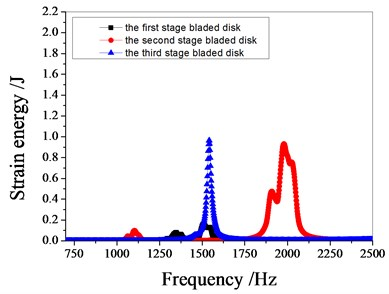f) 5 % mistuned bladed disk

Figs. 12-17 shows the distribution of strain energy of each blade disk at the resonance peak of tuned and mistuned bladed disk system.

It can be seen from Fig. 11 to Fig.17, the resonance frequency is around 1100 Hz or 1980 Hz, the strain energy is mainly concentrated in the second stage bladed disk, dominated by blade vibration, the resonance frequency is near 1980 Hz and the strain energy is large. the resonance frequency is around 1370 Hz or 1540 Hz, and the strain energy is mainly concentrated in the first stage bladed disk and the third stage blade disk, the resonance frequency is around 1370 Hz, the strain energy is small, and the vibration of the first stage bladed disk is mainly. the resonance frequency is around 1540 Hz, and the strain energy is large, mainly the third stage blade disk vibration. with the increase of mistuning standard deviation, the vibration energy of each stage of bladed disk decreases gradually.

Fig. 12Strain energy of tuned bladed disk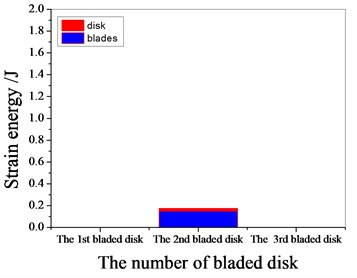a) 1097.7 Hz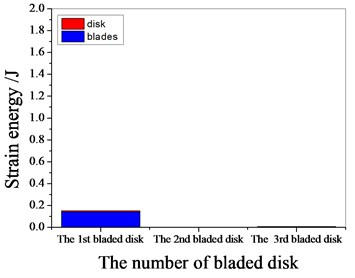b) 1373 Hz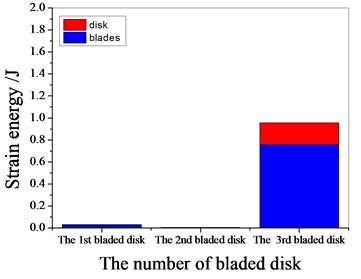c) 1541.7 Hz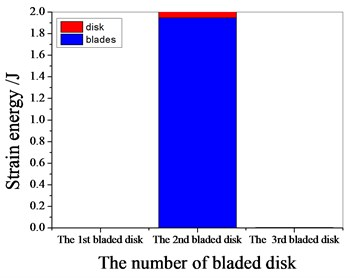d) 1978.3 Hz

Fig. 13Strain energy of 1 % mistuned bladed disk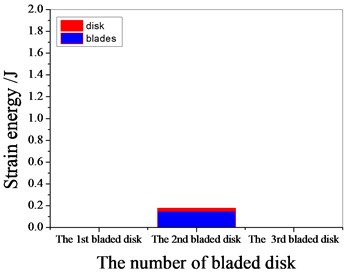a) 1097.7 Hz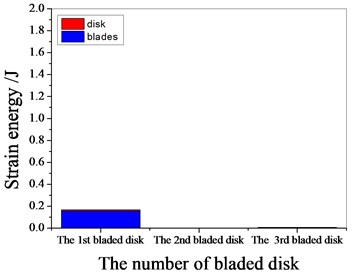b) 1373 Hz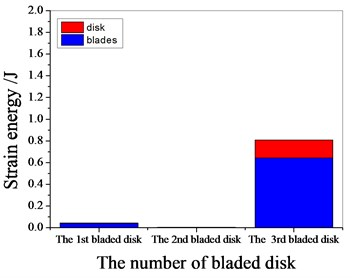c) 1541.7 Hz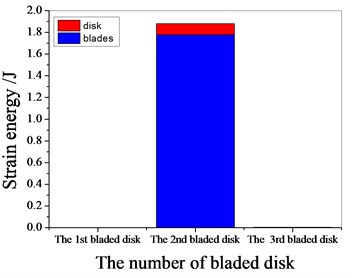d) 1978.3 Hz

Fig. 14Strain energy of 2 % mistuned bladed disk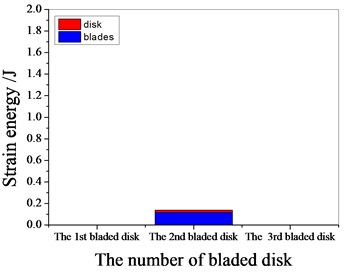a) 1101.4 Hz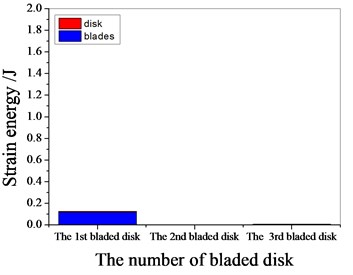b) 1369.65 Hz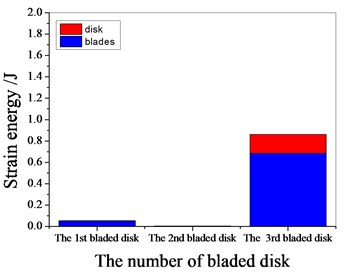c) 1539.85 Hz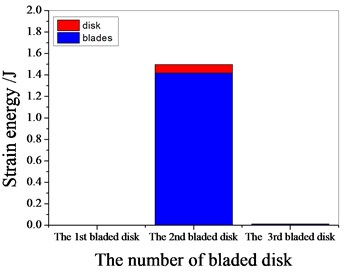d) 1980.15 Hz

Fig. 15Strain energy of 3 % mistuned bladed disk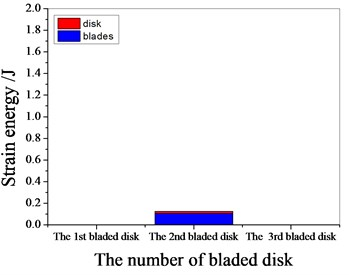a) 1094 Hz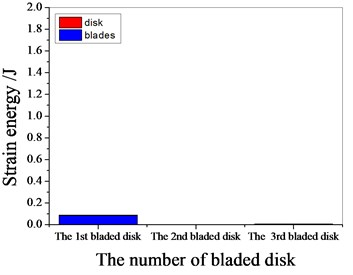b) 1367.8 Hz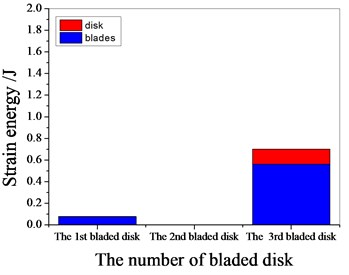c) 1538 Hz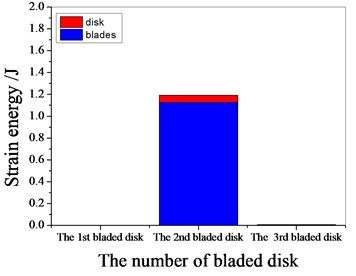d) 1987.55 Hz

Fig. 16Strain energy of 4 % mistuned bladed disk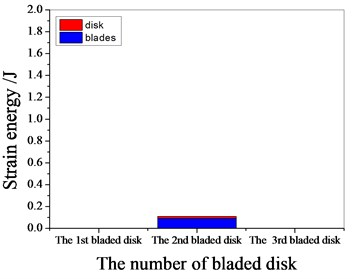a) 1099.55 Hz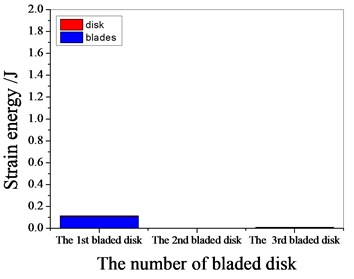b) 1365.95 Hz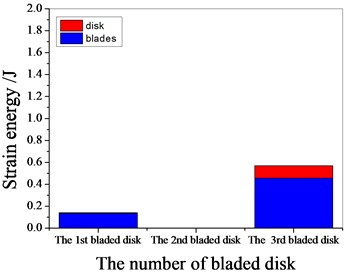c) 1532.45 Hz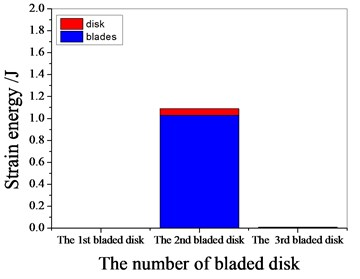d) 1974.6 Hz

Fig. 17Strain energy of 5 % mistuned bladed disk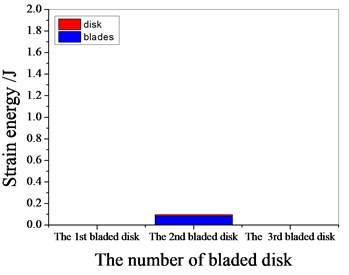a) 1103.25 Hz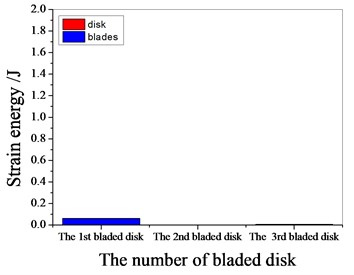b) 1373.35 Hz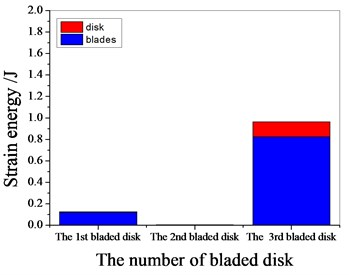c) 1541.7 Hz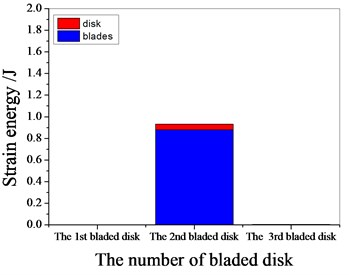d) 1980.15 Hz

## 5. Conclusions

The vibration characteristics of multistage mistuned bladed disk system were studied. Based on the substructure modal synthesis method, the finite element reduction model of multistage bladed disk was established, the accuracy of substructure model and integral bladed disk model is compared, and the influence of different modal truncation numbers on the calculation accuracy of substructure model is discussed. Through the modal analysis of multistage bladed disk, the effects of rotating softening effect and rotating speed on the dynamic frequency of blade disk system are analyzed. the modal shapes of typical orders of multistage bladed disks are obtained. Through the forced response analysis of the mistuned multistage bladed disk, the amplitude frequency characteristics of the tuned and mistuned bladed disk are obtained, the influence of different mistuned standard deviations on the vibration characteristics of the bladed disk system is discussed, and the vibration of the mistuned bladed disk is analyzed from the perspective of strain energy. The following points are concluded through modal analysis and vibration analysis:

1) with the increase of modal truncation number, the calculation accuracy of substructure model increases gradually.

2) the rotating speed has a significant influence on the dynamic frequency of multistage bladed disk system. with the increase of rotating speed, as a result the dynamic frequency increases.

3) the first-order bending vibration of the blade is the main form of the low-order vibration of the first 200 order multistage blade disk.

4) the maximum strain energy of each stage bladed disk system decreases with the increase of mistuning standard deviation.

#### Acknowledgements

This research was funded by project of the National Natural Science Foundation of China (No. 51805076, No. 51775093 and No. U1708255), Doctoral research initiation fund of Liaoning Provincial (2020-BS-156), Shenyang Youth Science and technology innovation talent project (RC200006) and Scientific research fund project of Liaoning Provincial Department of Education (LG202008), the construction plan of scientific research and innovation team of Shenyang Ligong University (SYLU202101), Comprehensive reform project of graduate education of Shenyang Ligong University (2021DSTD004, 2021PYPT006).